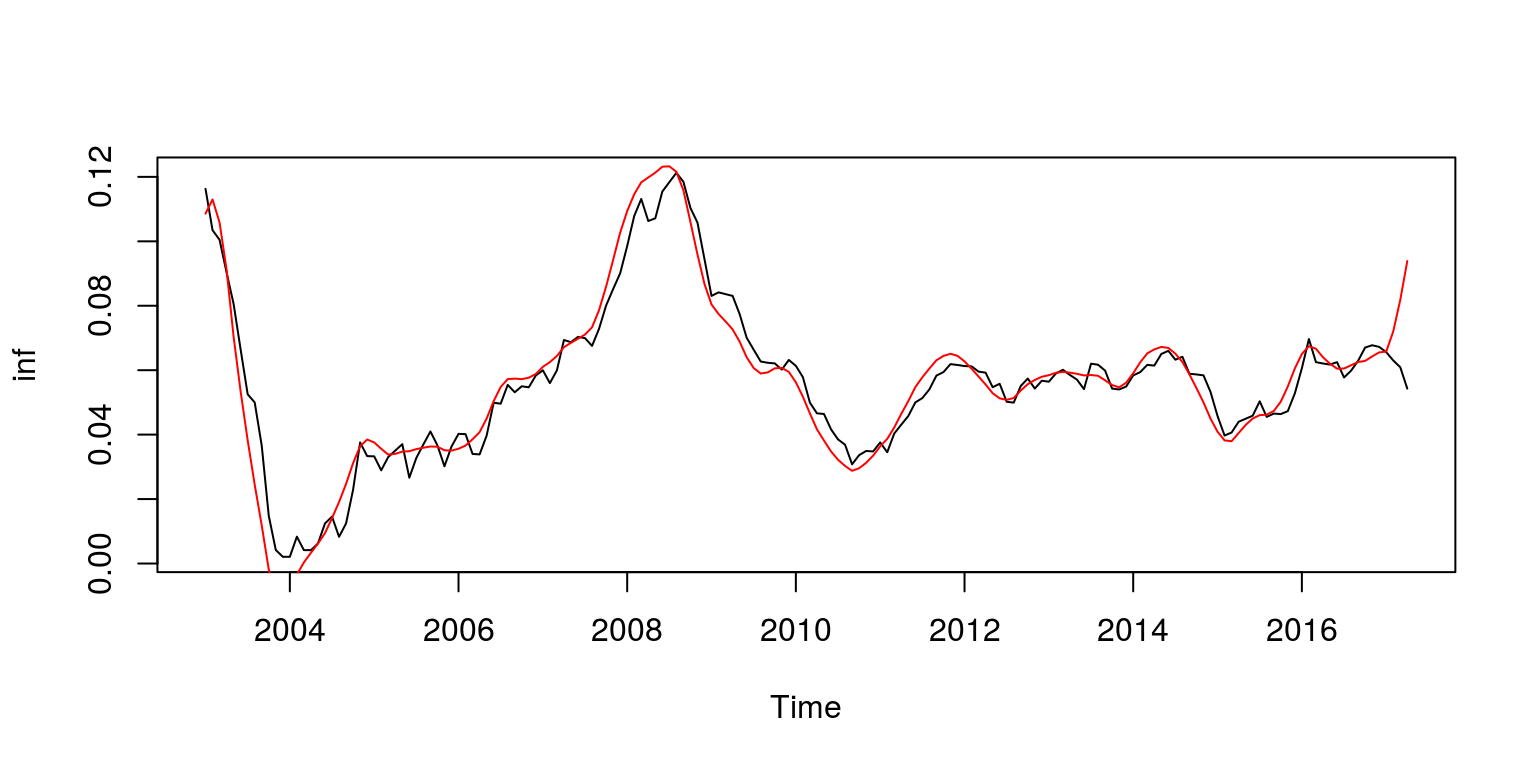# 1 Decomposing South African output

The first program for this session contains various filters that may be used to decompose a measure of South African output. This example is contained in the file `T5-decomp.R`. Once again, the first thing that we do is clear all variables from the current environment and close all the plots. This is performed with the following commands:

``````rm(list = ls())
graphics.off()``````

Thereafter, we will need to install the `tsm` package from my GitHub account, as well as the `mFilter` package that was written by one of my co-authors.

``````devtools::install_github("KevinKotze/tsm")
install.packages("mFilter", repos = "https://cran.rstudio.com/",
dependencies = TRUE)``````

The next step is to make sure that you can access the routines in these packages by making use of the `library` command.

``````library(tsm)
library(mFilter)``````

As noted previosuly, South African GDP data has been preloaded in the `tsm` package, where Real GDP is numbered KBP6006D. Hence, to retrieve the data from the package and create the object for the natural logarithm of GDP that will be stored as a time series in `gdp`, we execute the following commands:

``````dat <- sarb_quarter\$KBP6006D
dat.tmp <- log(na.omit(dat))
gdp <- ts(dat.tmp, start = c(1960, 2), frequency = 4)``````

To make sure that these calculations and extractions have been performed correctly, we inspect a plot of the data.

``plot(gdp)``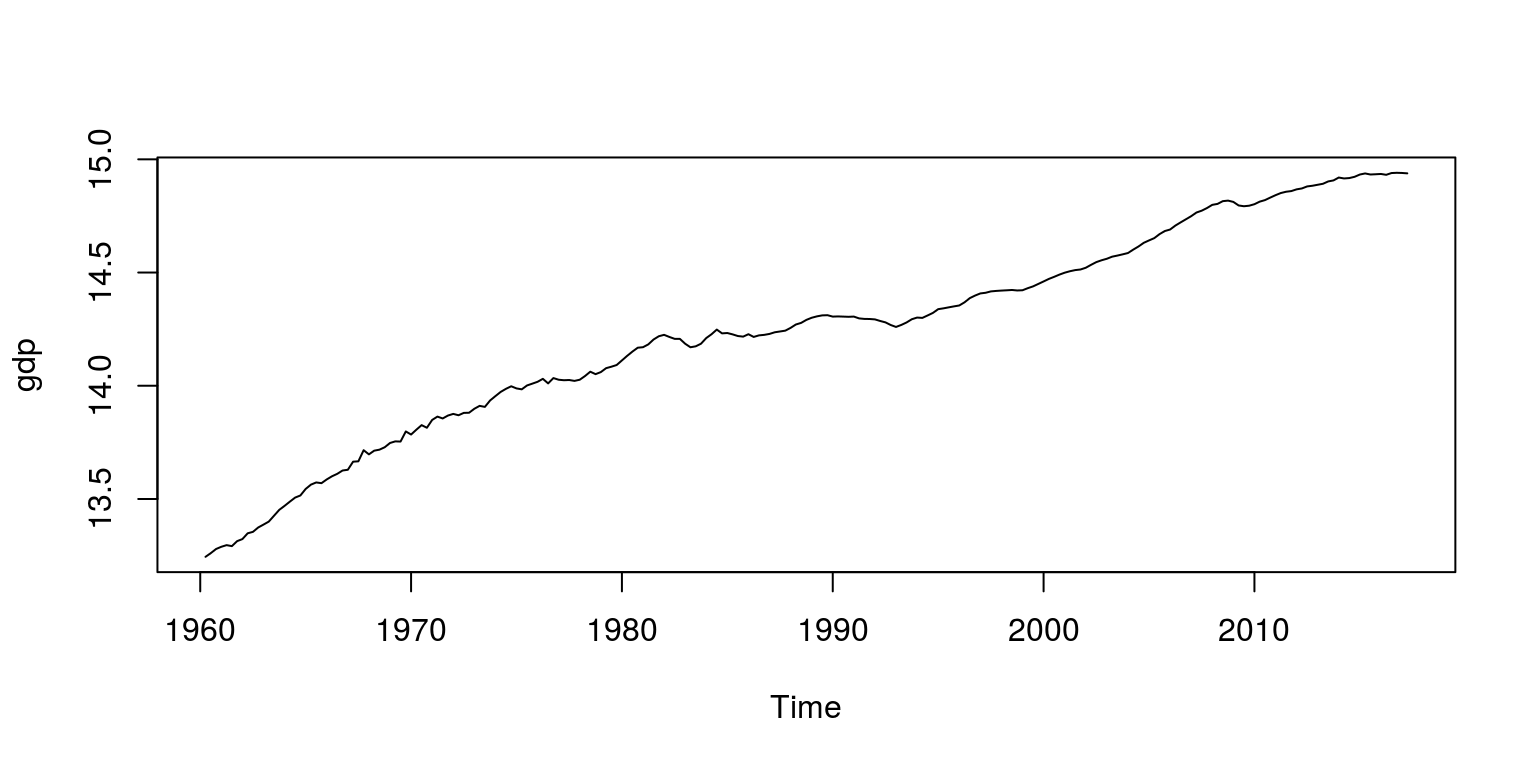## 1.2 Detrend data with a linear filter

To estimate a linear trend we can make use of a linear regression model that includes a time trend and a constant. To estimate such a model we make use of the `lm` command as follows.

``````lin.mod <- lm(gdp ~ time(gdp))
lin.trend <- lin.mod\$fitted.values  # fitted values pertain to time trend
linear <- ts(lin.trend, start = c(1960, 1), frequency = 4)  # create a time series variable for trend
lin.cycle <- gdp - linear  # cycle is the difference between the data and linear trend``````

The fitted values from the regression would then contain the information that pertains to the linear trend. These would need to be extracted from the model object `lin.mod` and in the above chunk we have allocated these values to the time series object `linear`. The cycle is then derived from subtracting the trend from the data.

We can then plot this result with the aid of the following commands, where the trend and the cycle are plotted on separate figures.

``````par(mfrow = c(1, 2), mar = c(2.2, 2.2, 1, 1), cex = 0.8)  # plot two graphs side by side and squash margins
plot.ts(gdp, ylab = "")  # first plot time series
lines(linear, col = "red")  # include lines over the plot
legend("topleft", legend = c("data", "trend"), lty = 1,
col = c("black", "red"), bty = "n")
plot.ts(lin.cycle, ylab = "")  # second plot for cycle
legend("topright", legend = c("cycle    "), lty = 1, col = c("black"),
bty = "n")``````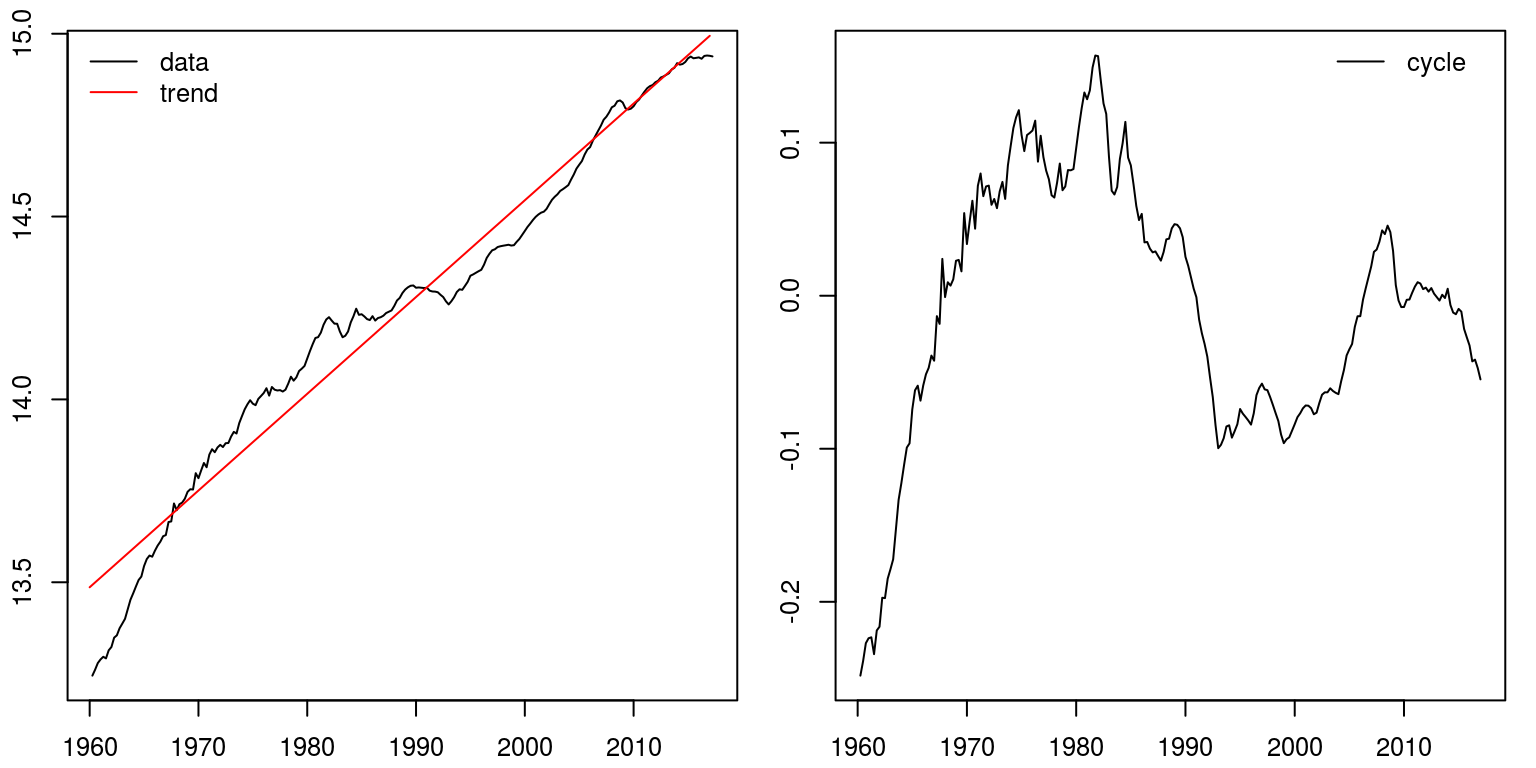## 1.3 Detrend data with the Hodrick-Prescott filter

To detrend this data with the popular Hodrick-Prescott filter we only need to make use of a single command. In this case we are setting the value for lambda equal to 1600, which is what has been suggested for quarterly data.

``````hp.decom <- hpfilter(gdp, freq = 1600, type = "lambda")

par(mfrow = c(1, 2), mar = c(2.2, 2.2, 1, 1), cex = 0.8)
plot.ts(gdp, ylab = "")  # plot time series
lines(hp.decom\$trend, col = "red")  # include HP trend
legend("topleft", legend = c("data", "HPtrend"), lty = 1,
col = c("black", "red"), bty = "n")
plot.ts(hp.decom\$cycle, ylab = "")  # plot cycle
legend("topleft", legend = c("HPcycle"), lty = 1, col = c("black"),
bty = "n")``````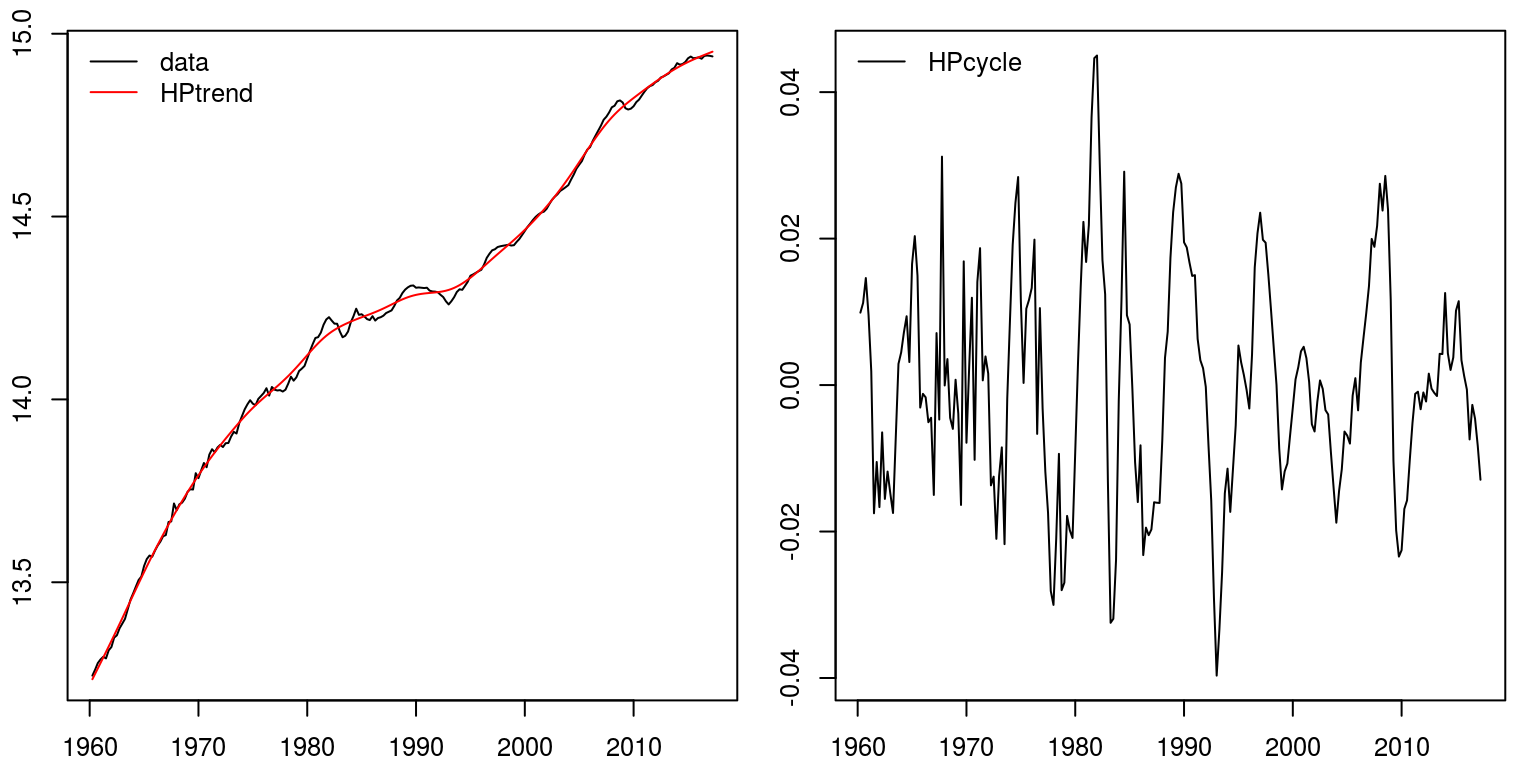Note that this would appear to provide a more accurate representation of what we understand of South Africas economic performance.

## 1.4 Detrend data with the Baxter-King filter

To make use of the Baxter-King band pass filter we can employ a similar command to what was used above. In this case we need to specify the frequency band for the cycle, where the upper limit is set at 32 and lower limit is set at 6.

``````bp.decom <- bkfilter(gdp, pl = 6, pu = 32)

par(mfrow = c(1, 2), mar = c(2.2, 2.2, 1, 1), cex = 0.8)
plot.ts(gdp, ylab = "")
lines(bp.decom\$trend, col = "red")
legend("topleft", legend = c("data", "BPtrend"), lty = 1,
col = c("black", "red"), bty = "n")
plot.ts(bp.decom\$cycle, ylab = "")
legend("topleft", legend = c("BPcycle"), lty = 1, col = c("black"),
bty = "n")``````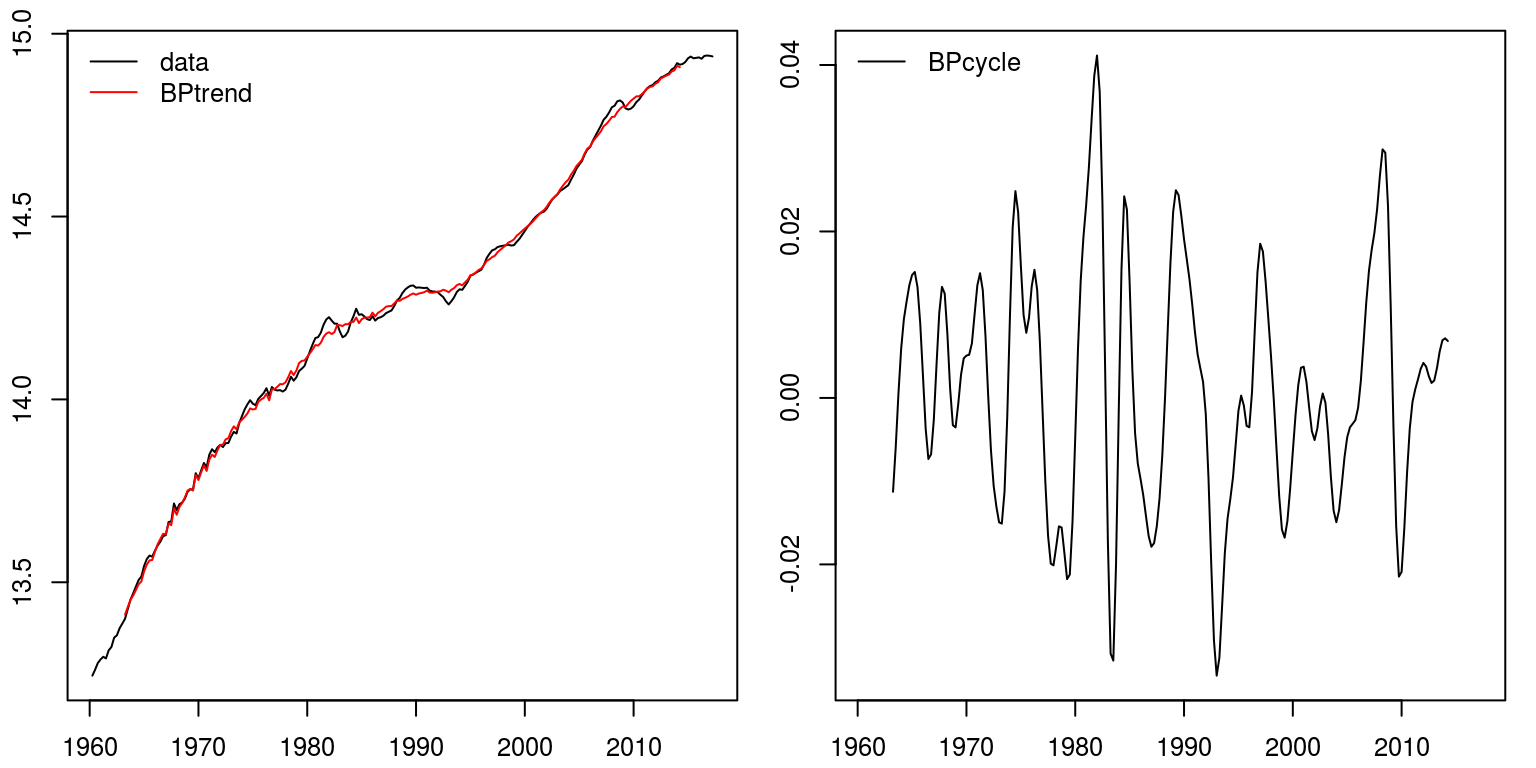Once again this seems to provide a fairly accurate representation of the cyclical nature of economic activity in South Africa. Note also that the representation of the cycle is much smoother than what was provided previously, as the noise is not included with the cycle.

## 1.5 Detrend data with the Christiano-Fitzgerald filter

This filter is very similar in nature to what was provided above. In addition, it also generates a highly similar result to that of the Baxter-King filter.

``````cf.decom <- cffilter(gdp, pl = 6, pu = 32, root = TRUE)

par(mfrow = c(1, 2), mar = c(2.2, 2.2, 1, 1), cex = 0.8)
plot.ts(gdp, ylab = "")
lines(cf.decom\$trend, col = "red")
legend("topleft", legend = c("data", "CFtrend"), lty = 1,
col = c("black", "red"), bty = "n")
plot.ts(cf.decom\$cycle, ylab = "")
legend("topleft", legend = c("CFcycle"), lty = 1, col = c("black"),
bty = "n")``````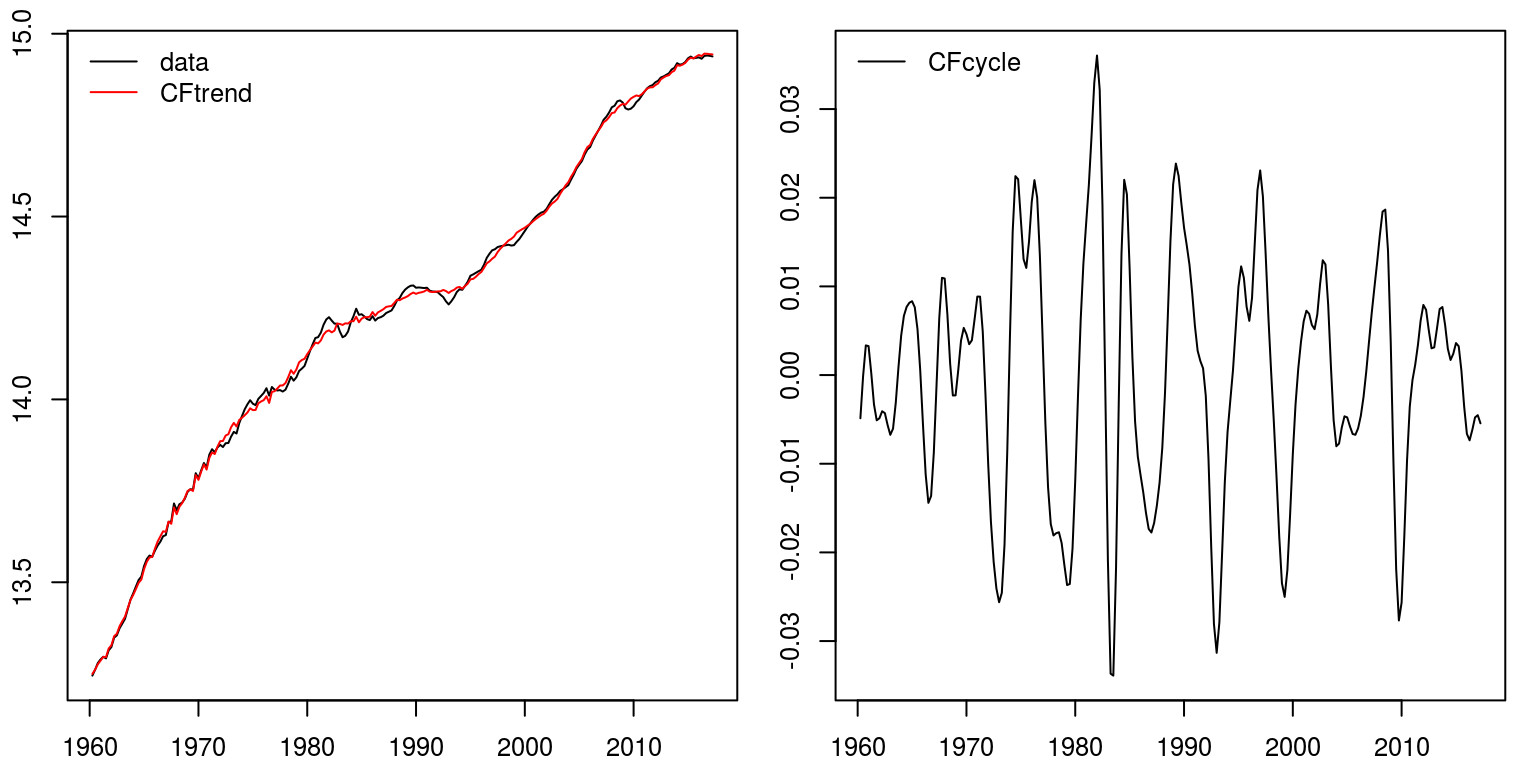## 1.6 Detrend data with the Beveridge-Nelson decomposition

To decompose output into a stochastic trend and stationary cycle, we can employ the Beveridge-Nelson decomposition. When employing this technique we would need to specify the number of lags that would pertain to the stationary component. In the example that I’ve included below, I’ve assume eight lags.

``````bn.decomp <- bnd(gdp, nlag = 8)  # apply the BN decomposition that creates dataframe

bn.trend <- ts(bn.decomp[, 1], start = c(1960, 1), frequency = 4)  # first column contains trend
bn.cycle <- ts(bn.decomp[, 2], start = c(1960, 1), frequency = 4)  # second column contains cycle

par(mfrow = c(1, 2), mar = c(2.2, 2.2, 1, 1), cex = 0.8)
plot.ts(gdp, ylab = "")
lines(bn.trend, col = "red")
legend("topleft", legend = c("data", "BNtrend"), lty = 1,
col = c("black", "red"), bty = "n")
plot.ts(bn.cycle, ylab = "")
legend("topleft", legend = c("BNcycle"), lty = 1, col = c("black"),
bty = "n")``````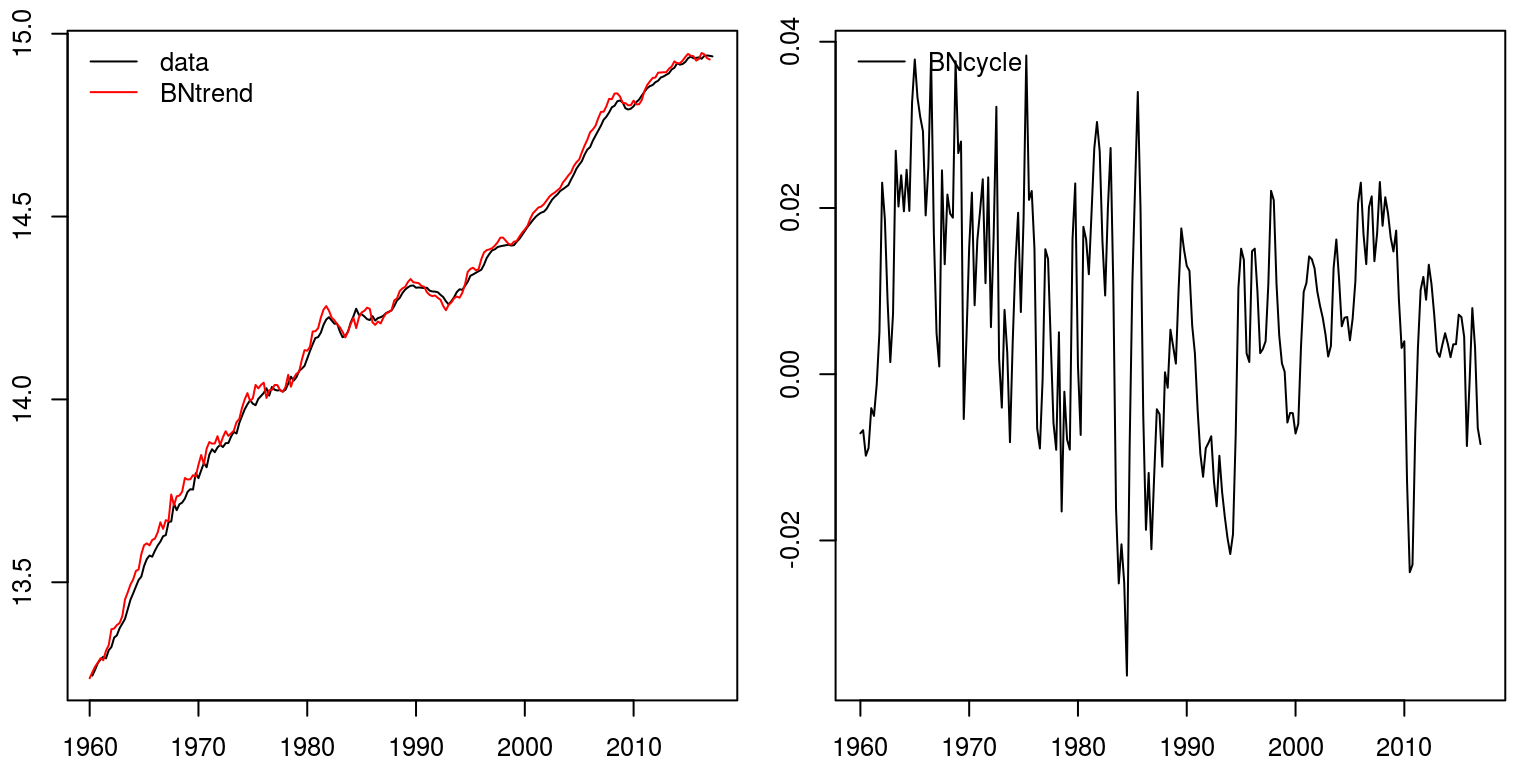## Comparing different measures of the cycle

We can then combine all these results on a single graph to consider the respective similarities and differences. In this case, I’ve created a time series union, `ts.union`, but I could have also plotted a single series, before using the `lines` command to plot successive plots on top of that.

``````comb <- ts.union(lin.cycle, hp.decom\$cycle, bp.decom\$cycle,
cf.decom\$cycle, bn.cycle)

par(mfrow = c(1, 1), mar = c(2.2, 2.2, 2, 1), cex = 0.8)
plot.ts(comb, ylab = "", plot.type = "single", col = c("blue",
"red", "darkgrey", "sienna", "darkgreen"))
legend("topleft", legend = c("linear", "hp-filter", "bp-filter",
"cf-filter", "bn-decomp"), lty = 1, col = c("blue",
"red", "darkgrey", "sienna", "darkgreen"), bty = "n")``````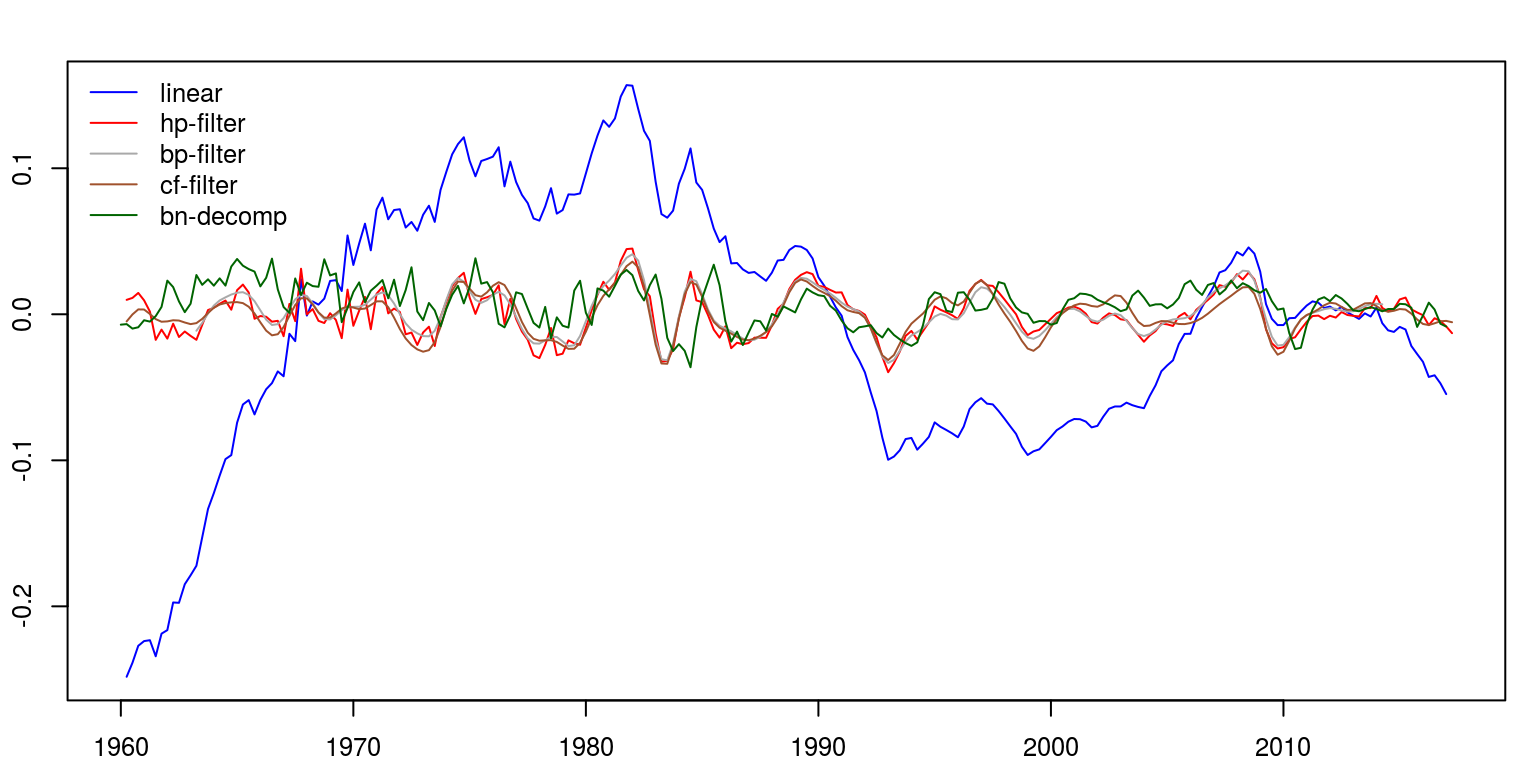# 2 Spectral decompositions

Before we consider the use of spectral techniques it would be a good idea to clear all variables from the current environment and close all the plots.

``````rm(list = ls())
graphics.off()``````

The next step is to make sure that you can access the routines in these packages by making use of the `library` command.

``````library(tsm)
library(TSA)
library(mFilter)``````

In class we simulated some data and decomposed it using spectral techniques. To replicate this example, we could generate values for three time series variables, which are then combined to form a single variable.

``````no.obs <- 100
t <- seq(1, no.obs, 1)
w <- c(6/no.obs, 30/no.obs, 40/no.obs)

x1 <- 2 * cos(2 * pi * t * w) + 3 * sin(2 * pi * t *
w)  # frequency 6 cycles in no.obs points
x2 <- 4 * cos(2 * pi * t * w) + 5 * sin(2 * pi * t *
w)  # frequency 10 cycles in no.obs points
x3 <- 6 * cos(2 * pi * t * w) + 7 * sin(2 * pi * t *
w)  # frequency 40 cycles in no.obs points
y <- x1 + x2 + x3``````

To look at the behaviour of these variables we can plot them on a separate axes.

``````par(mfrow = c(2, 2), mar = c(2.2, 2.2, 2, 1), cex = 0.8)
plot(x1, type = "l", main = "x1")
plot(x2, type = "l", main = "x2")
plot(x3, type = "l", main = "x3")
plot(y, type = "l", main = "y")``````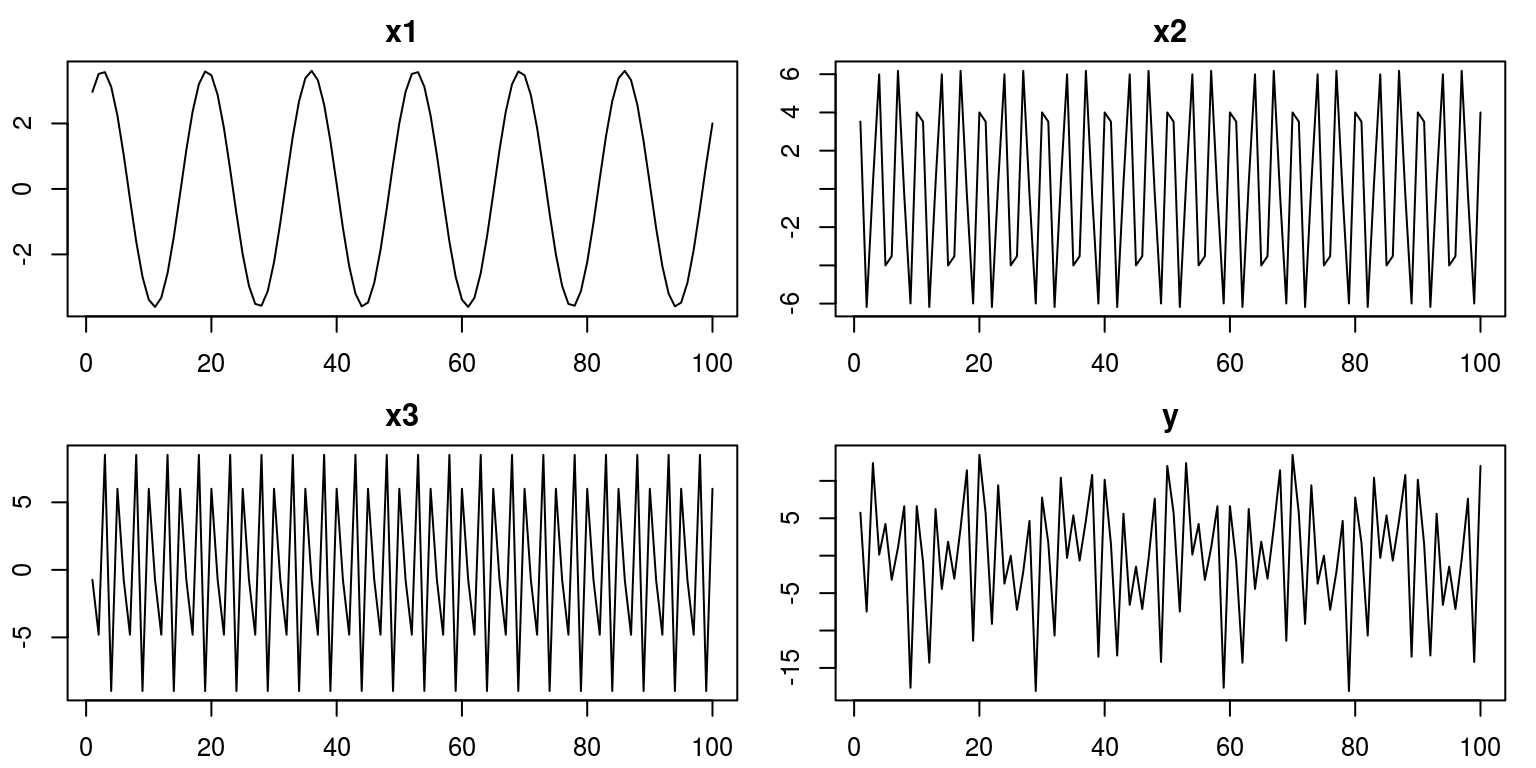Thereafter, we could use the periodogram to consider the properties of each of these time series variables.

``periodogram(x1, main = "x1", col = "red")``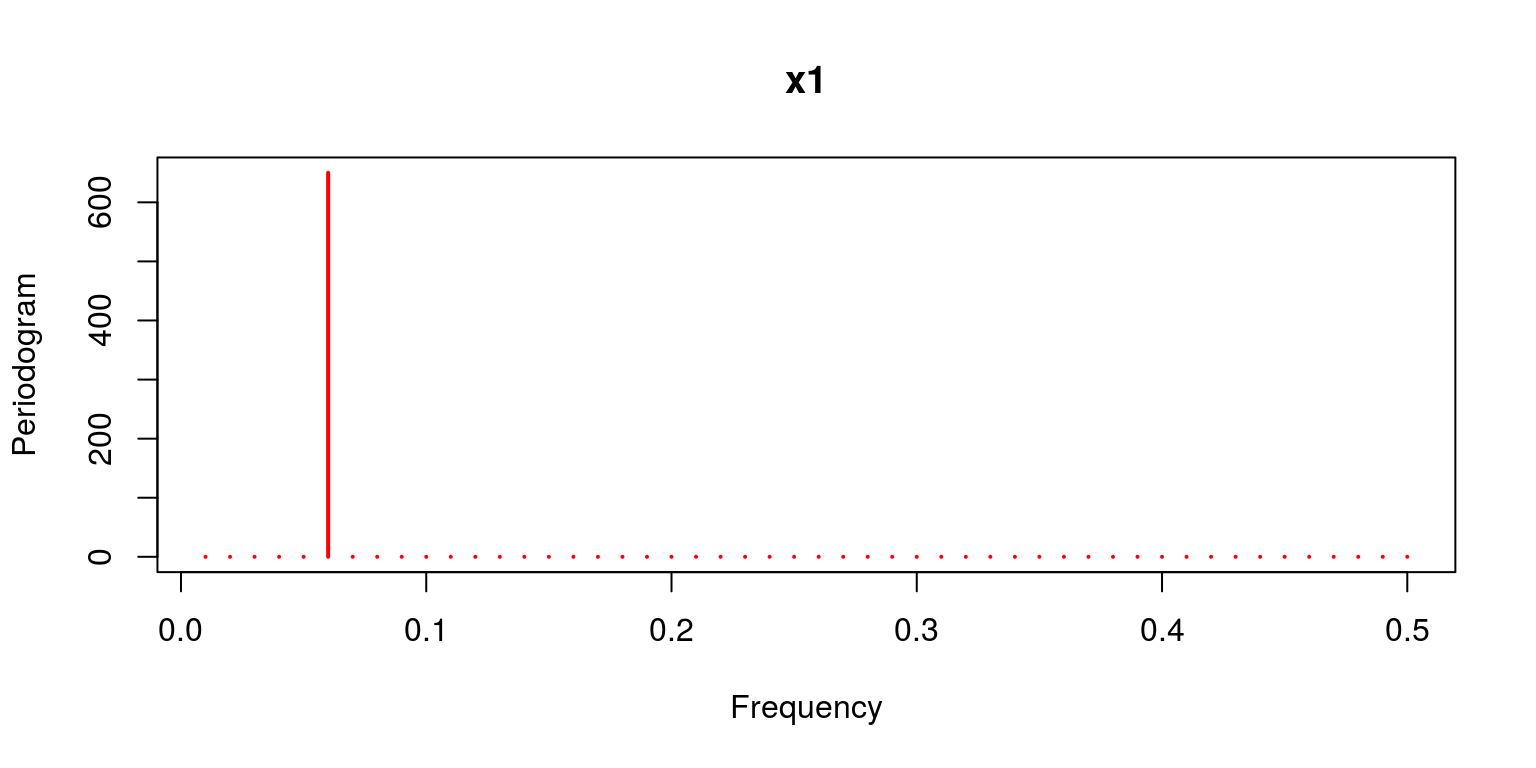``periodogram(x2, main = "x2", col = "red")``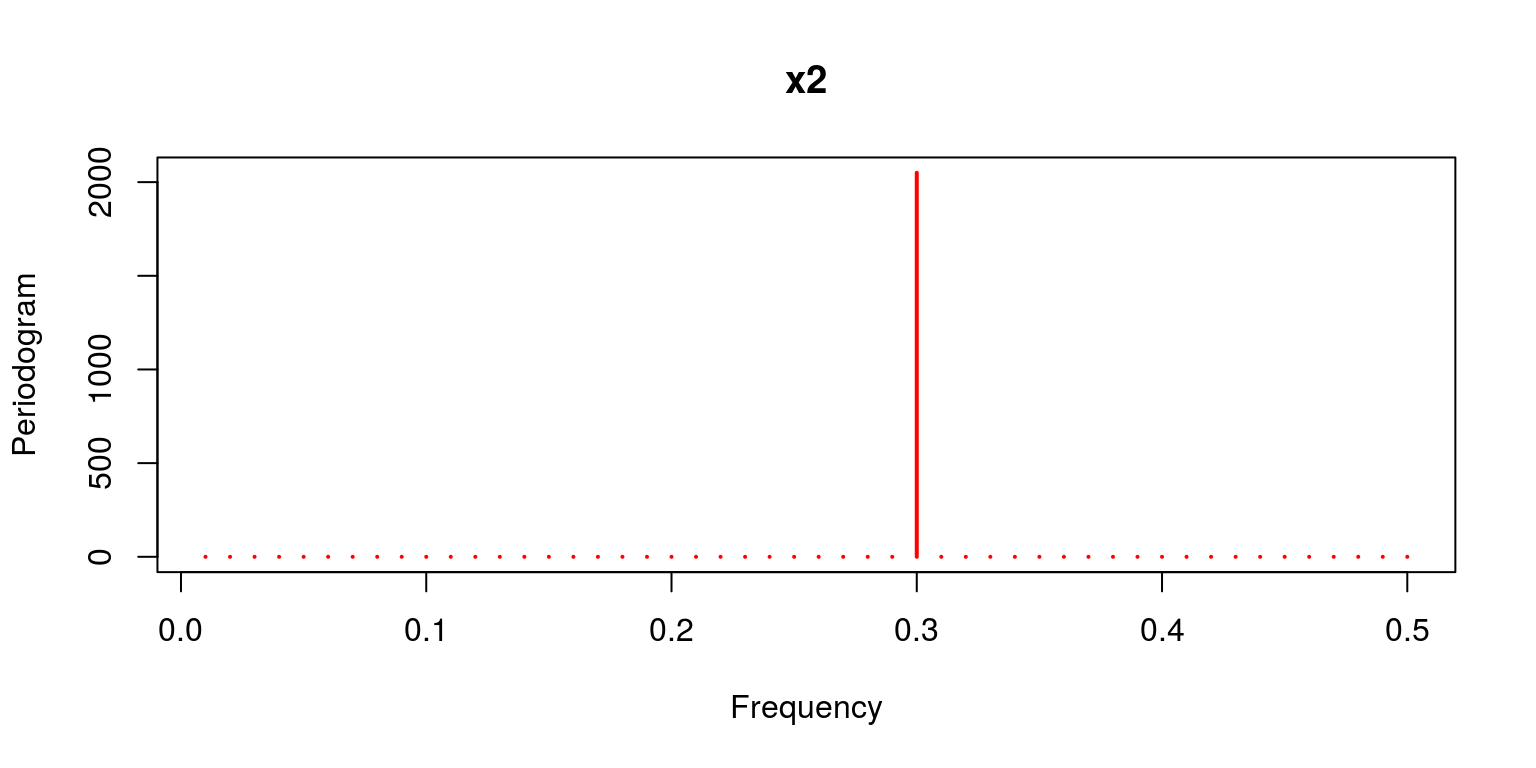``periodogram(x3, main = "x3", col = "red")``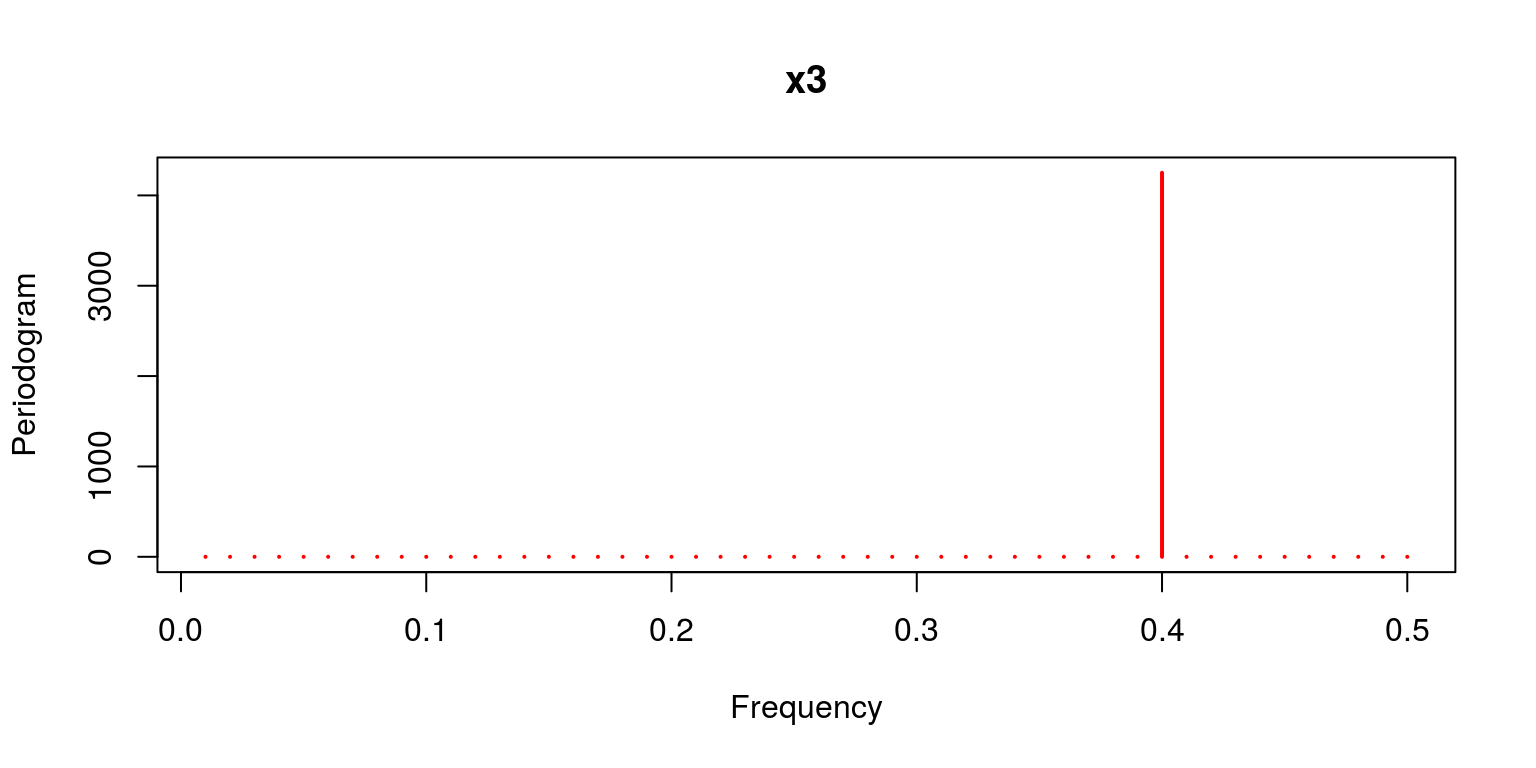``periodogram(y, main = "y", col = "red")``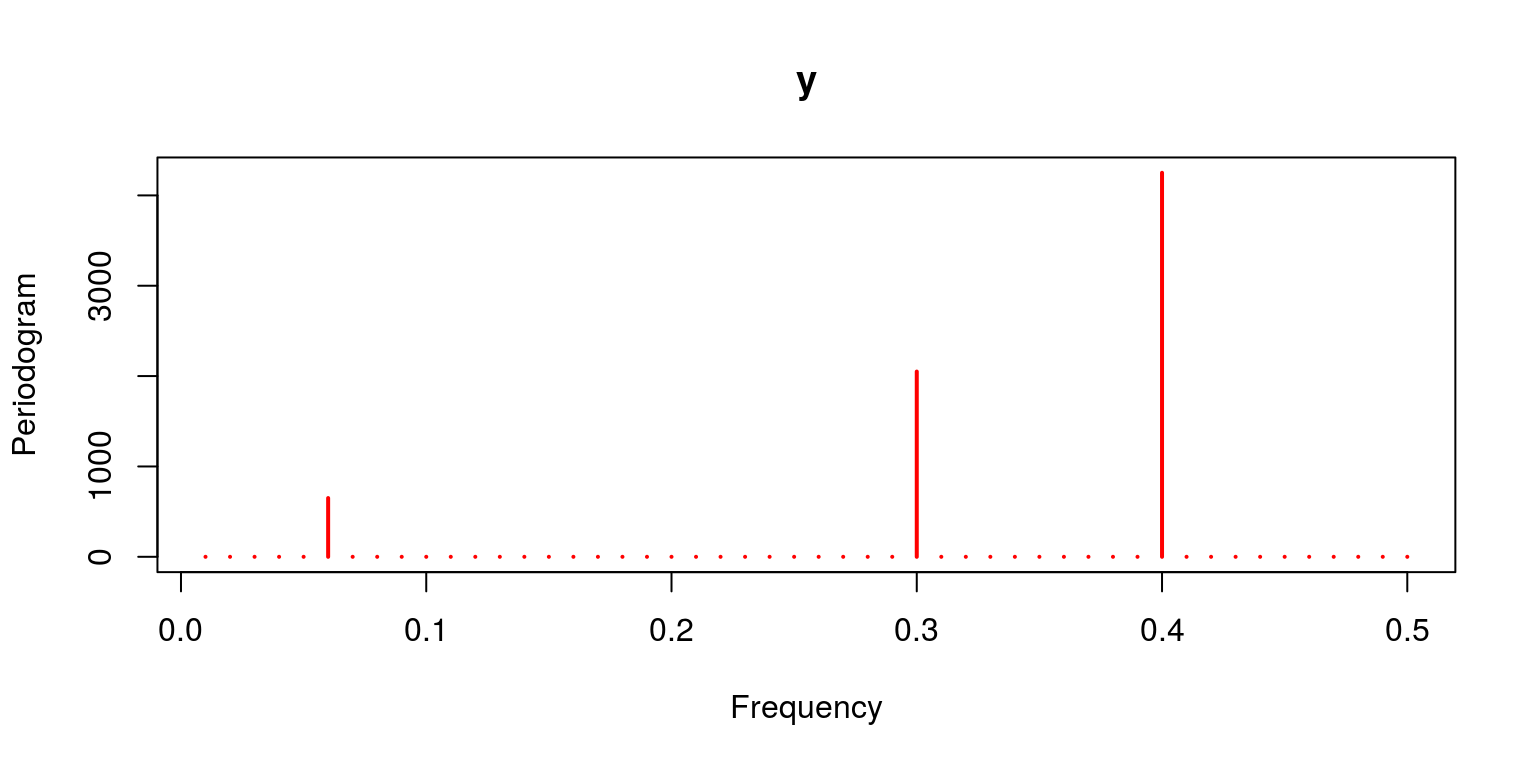We could of course make use of a filter to remove some of the unwanted components from the aggregate time series variable. To do so we could apply the Christiano-Fitzgerald filter with a relatively narrow upper and lower bound. Thereafter we use the periodogram that is applied to information relating to the cycle, to investigate whether it successfully excluded some of the frequency components.

``````ybp <- cffilter(y, pl = 16, pu = 20)
par(mfrow = c(1, 1))
periodogram(ybp\$cycle, col = "red")``````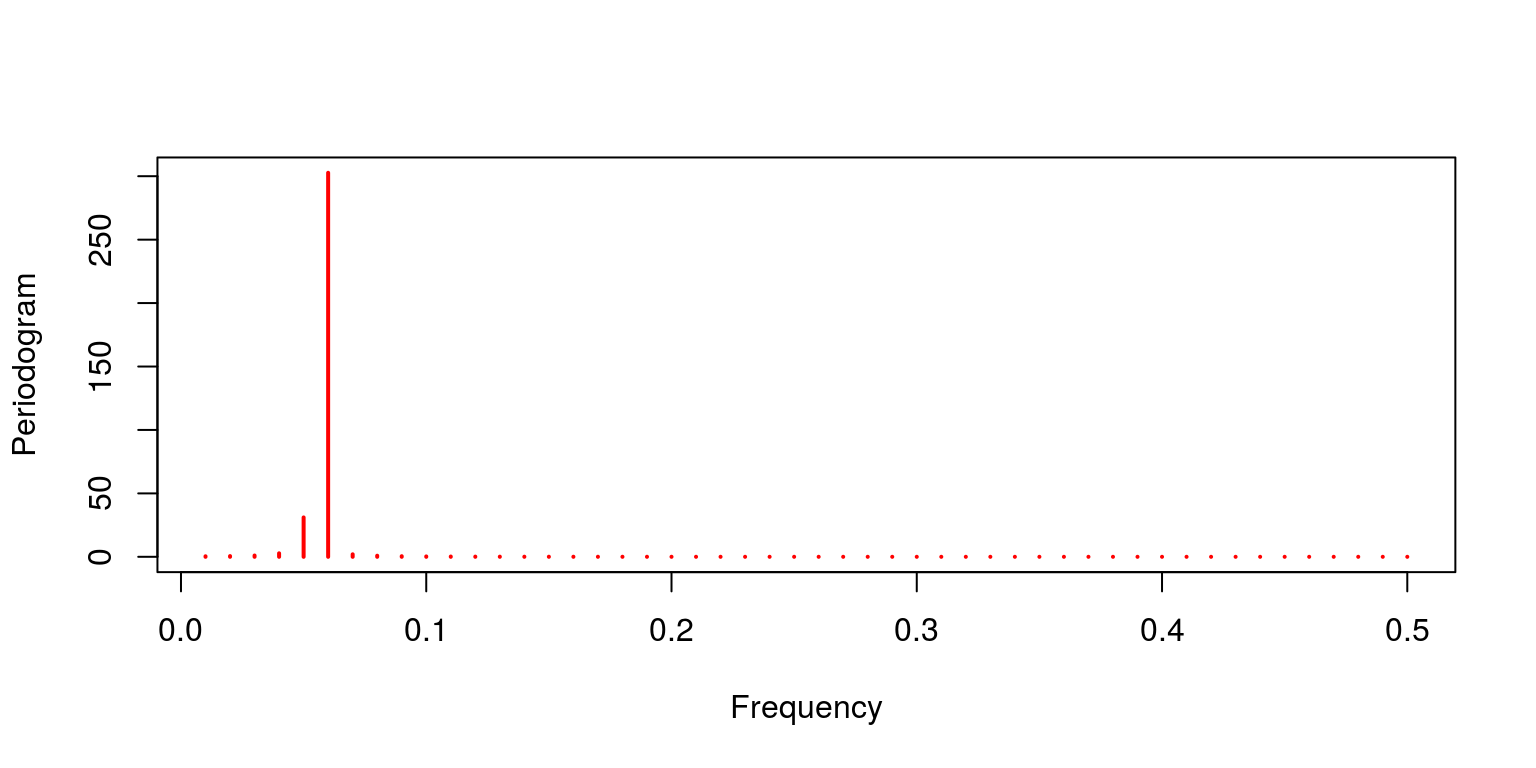This result would suggest that the filter has excluded most of the higher frequency components. To see how this cycle relates to the previous data we plot the cyclical information that passed through the filter onto the slow moving component. In addition, we also plot this result on the variable for the combined cycles.

``````par(mfrow = c(1, 2), mar = c(2.2, 2.2, 2, 1), cex = 0.8)
plot(x1, type = "l", lty = 1)
lines(ybp\$cycle, lty = 3, lwd = 3, col = "red")
legend("topleft", legend = c("X1", "Filtered     "), lty = c(1,
3), col = c("black", "red"), bty = "n")

plot(y, type = "l", lty = 1)
lines(ybp\$cycle, lty = 3, lwd = 3, col = "red")
legend("topleft", legend = c("Actual", "Filtered     "),
lty = c(1, 3), col = c("black", "red"), bty = "n")``````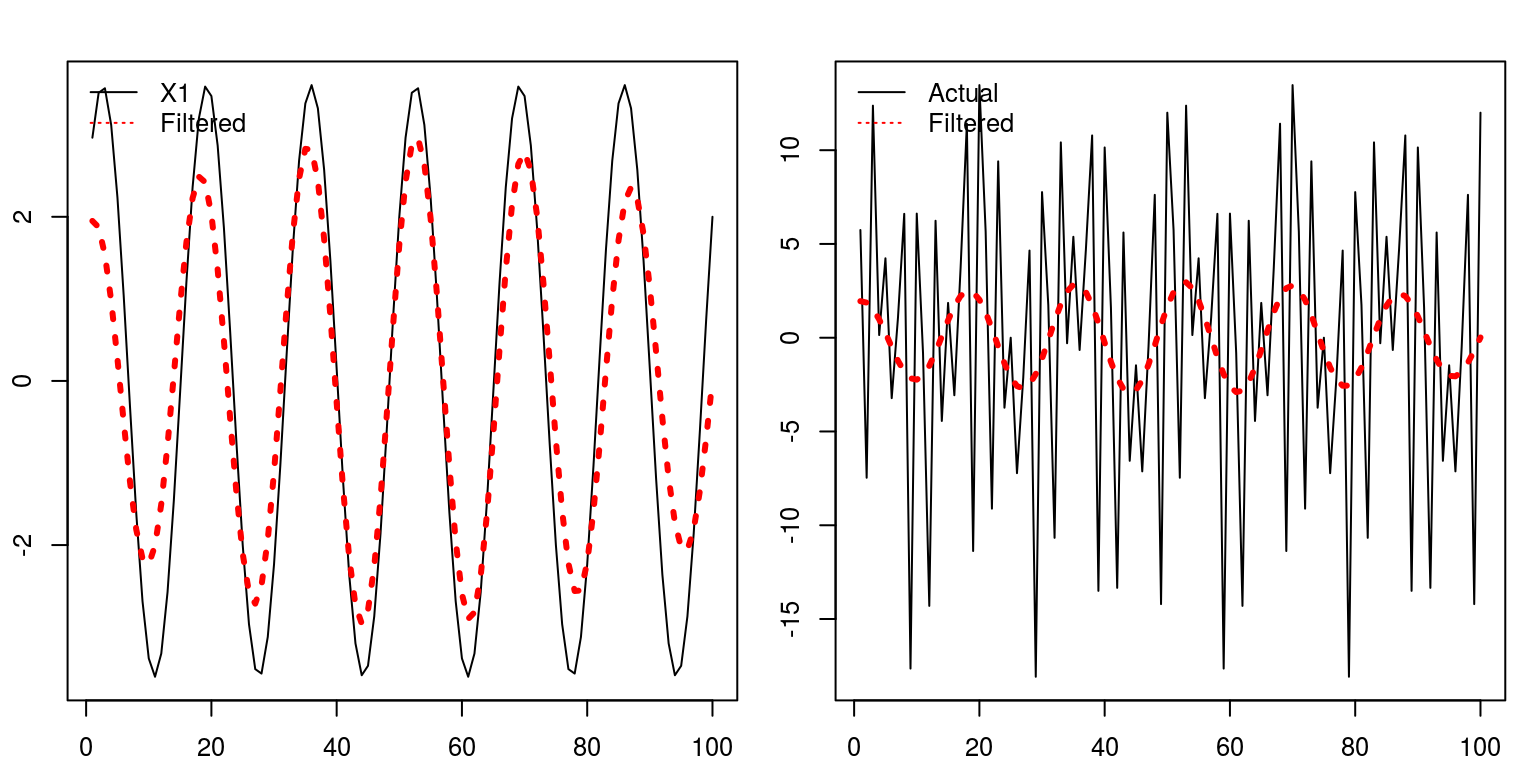In both cases it would appear to do a reasonable job of characterising the trend in the process.

## 2.1 Spectral decompositions on the South African business cycle

To consider how these spectral decompositions may be used in practice we can now consider the use of these techniques when applied to various characterisations of the South African business cycle. It would be useful to start this application with a clean workspace and plot window.

``````rm(list = ls())
graphics.off()``````

The next step would be to run all the filters that were applied to identify the different measures of the South African business cycle. Hence, to run the previous program we can use the `source` command, where we include the name of the file.

``source("T5_decomp.R")``

Let us now apply a periodogram to each measure of the business cycle.

``periodogram(lin.cycle, main = "Linear", col = "red")``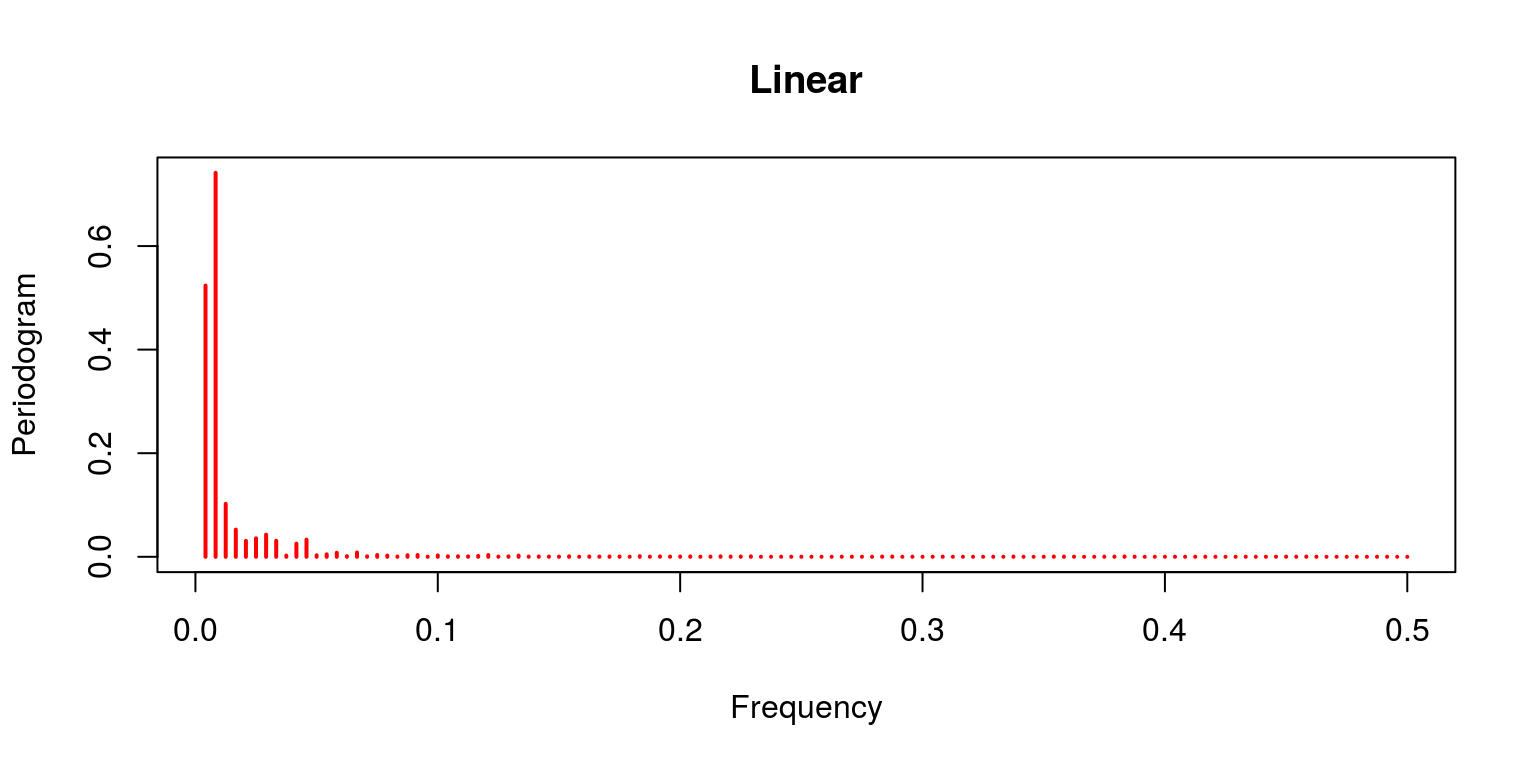``periodogram(hp.decom\$cycle, main = "Hodrick-Prescott", col = "red")``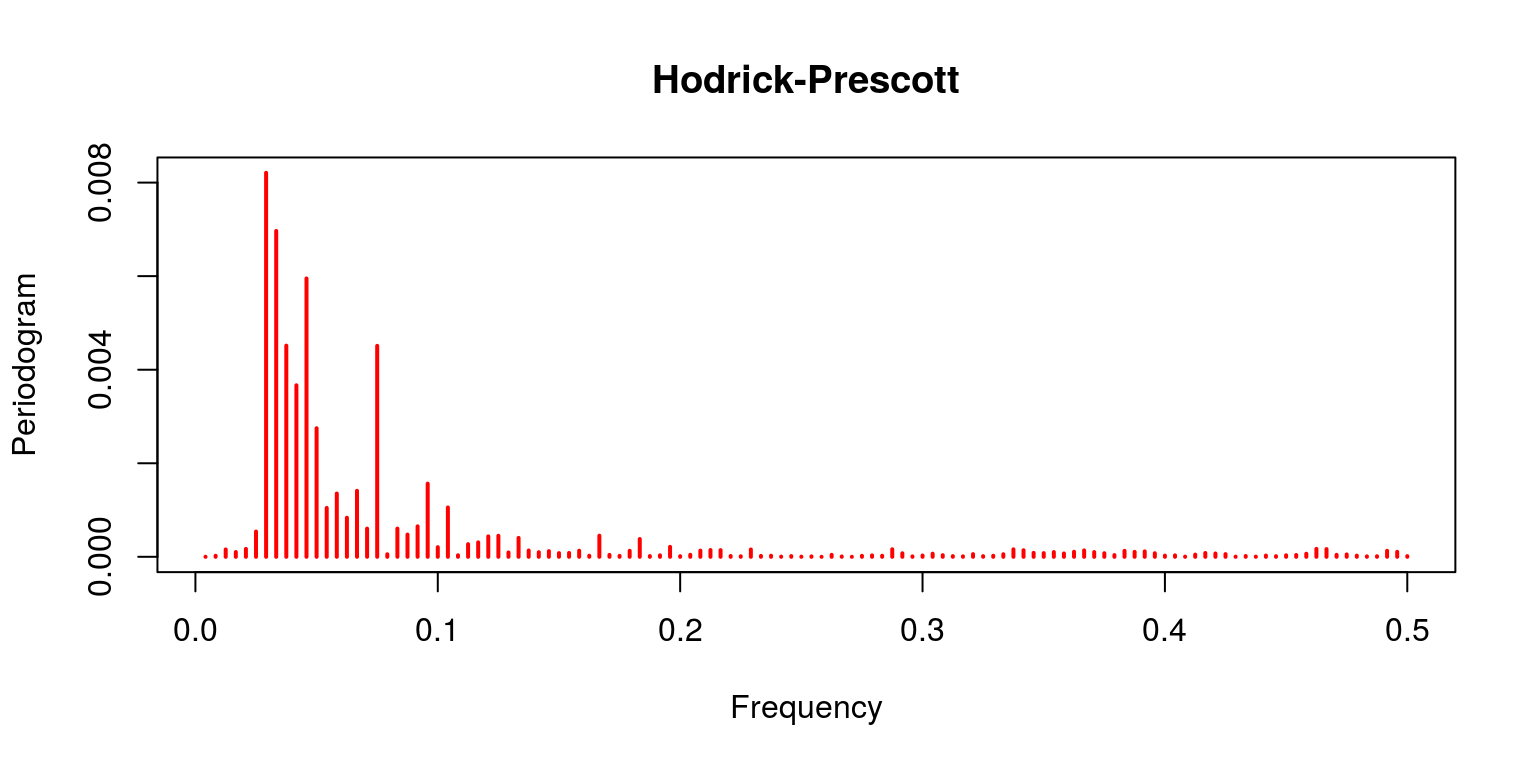``````periodogram(bp.decom\$cycle[13:(length(bp.decom\$cycle) -
12)], main = "Band-Pass", col = "red")``````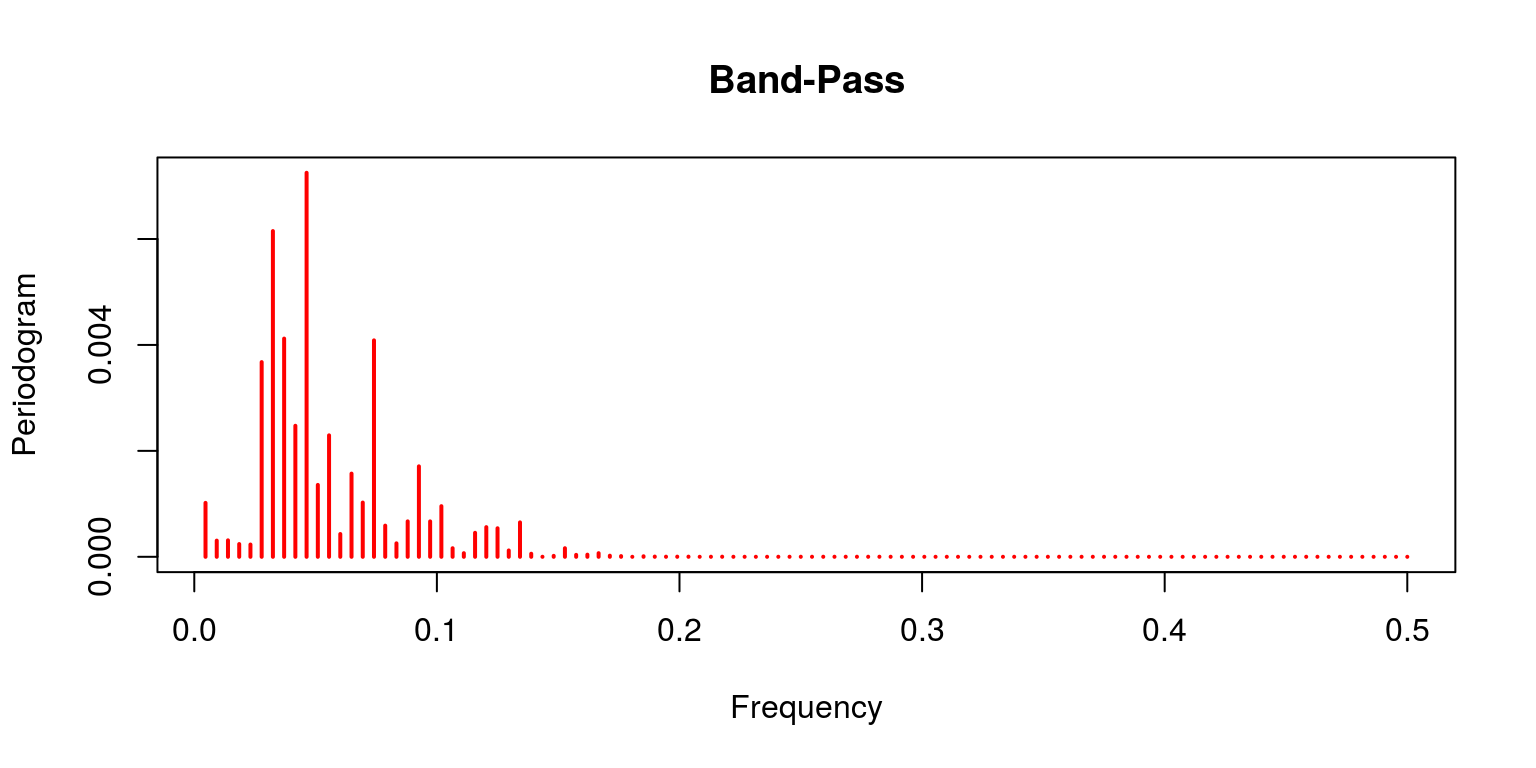``````periodogram(cf.decom\$cycle, main = "Christiano-Fitzgerald",
col = "red")``````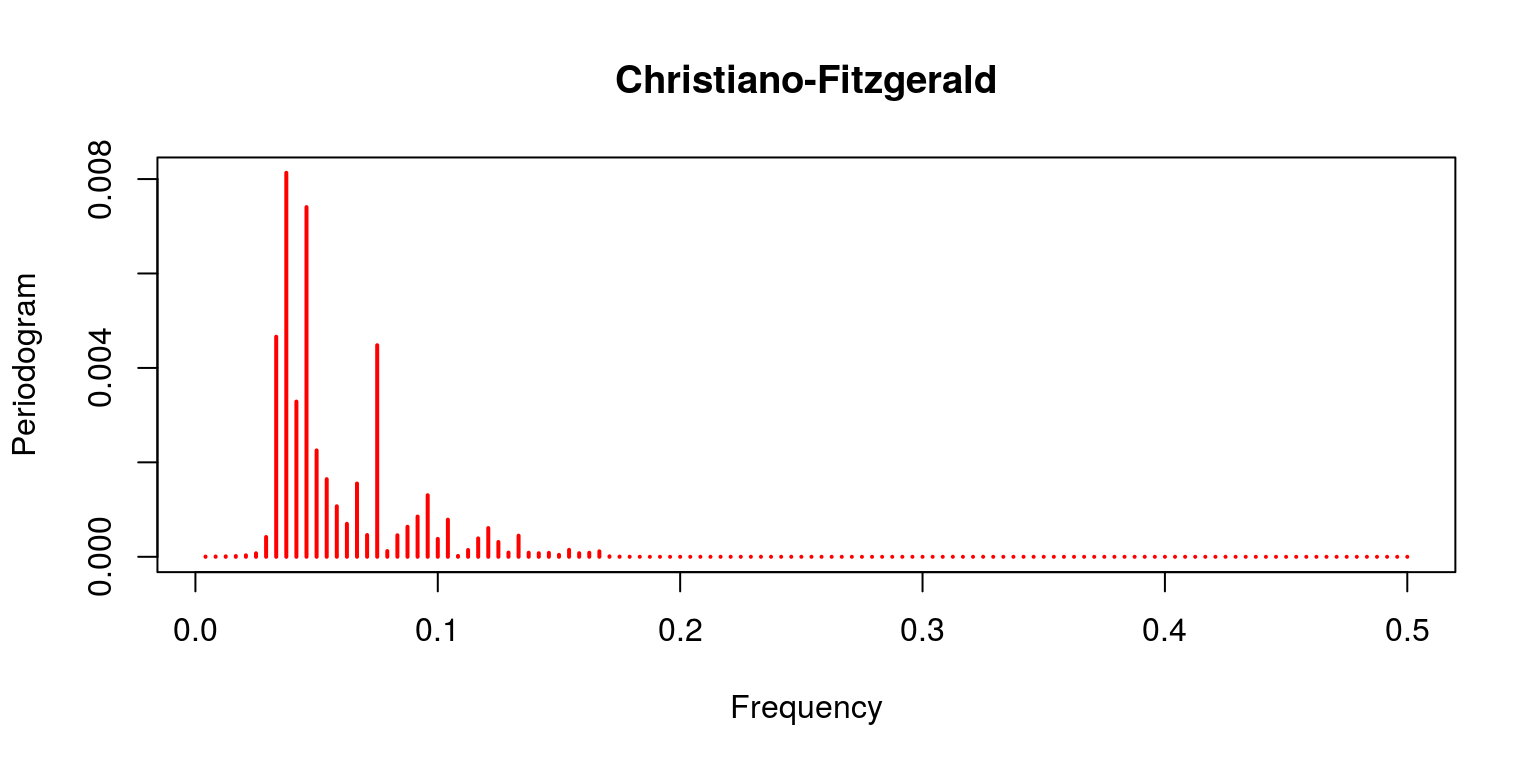``periodogram(bn.cycle, main = "Beveridge-Nelson", col = "red")``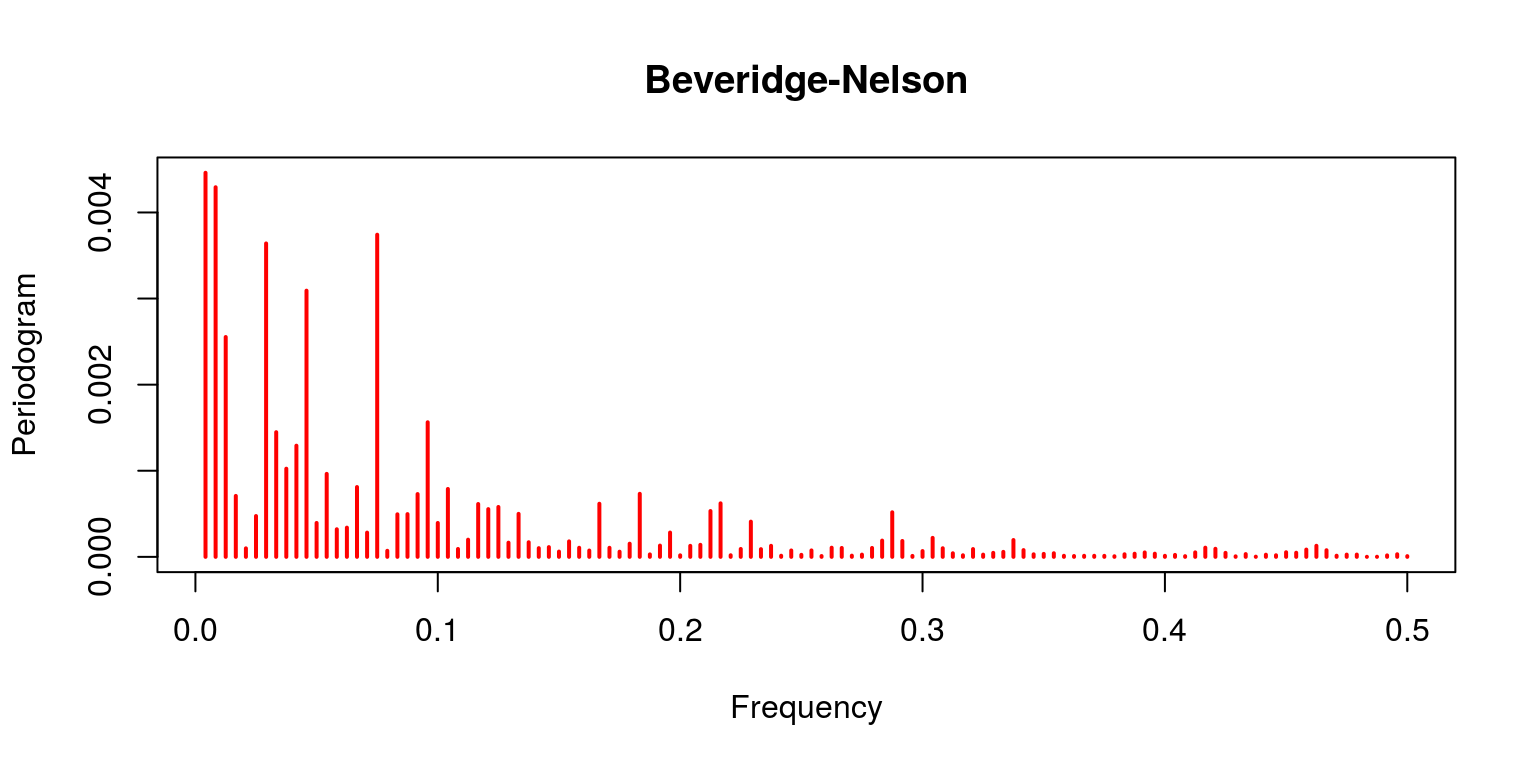Note that the linear filter provides a poor result as the trend clearly dominates (which is not what should be expected of the cycle). This is contrast with the characterisation of the Hodrick-Prescott filter, where the trending information has been removed. This is also the case for both the Band-Pass filters of Baxter & King and Christiano & Fitzgerald. In both of these cases the noise has also been removed. The final result pertains to the Beveridge-Nelson decomposition, where we note that the cycle includes a great deal of the trend and a large element of noise.

# 3 Wavelet decompositions

To provide an example of the wavelet decomposition we will apply the technique to data for South African inflation. This would allow use to derive an alternative measure for trend in the process, which may be considered to represent core inflation. Note that this technique may be applied to data of any integration order so we do not need to firstly consider the integration order of the variables. Once again, it would be useful to start with a clean workspace and plot window.

``````rm(list = ls())
graphics.off()``````

In this case we will make use of the `waveslim` and `tsm` packages.

``````library(tsm)
library(waveslim)``````

We will then make of monthly data for the consumer price index, which is contained the SARB quarterly bulletin release. The numeric for this variable is `KBP7170N` and the data extends back to 2002. To calculate a year-on-year measure of inflation we then make use of the `diff` and `lag` commands.

``````dat <- sarb_month\$KBP7170N
cpi <- ts(na.omit(dat), start = c(2002, 1), frequency = 12)
inf.yoy <- diff(cpi, lag = 12)/cpi[-1 * (length(cpi) - 11):length(cpi)]``````

To ensure that all these variable transformations have been performed correctly we plot the data.

``plot(inf.yoy)``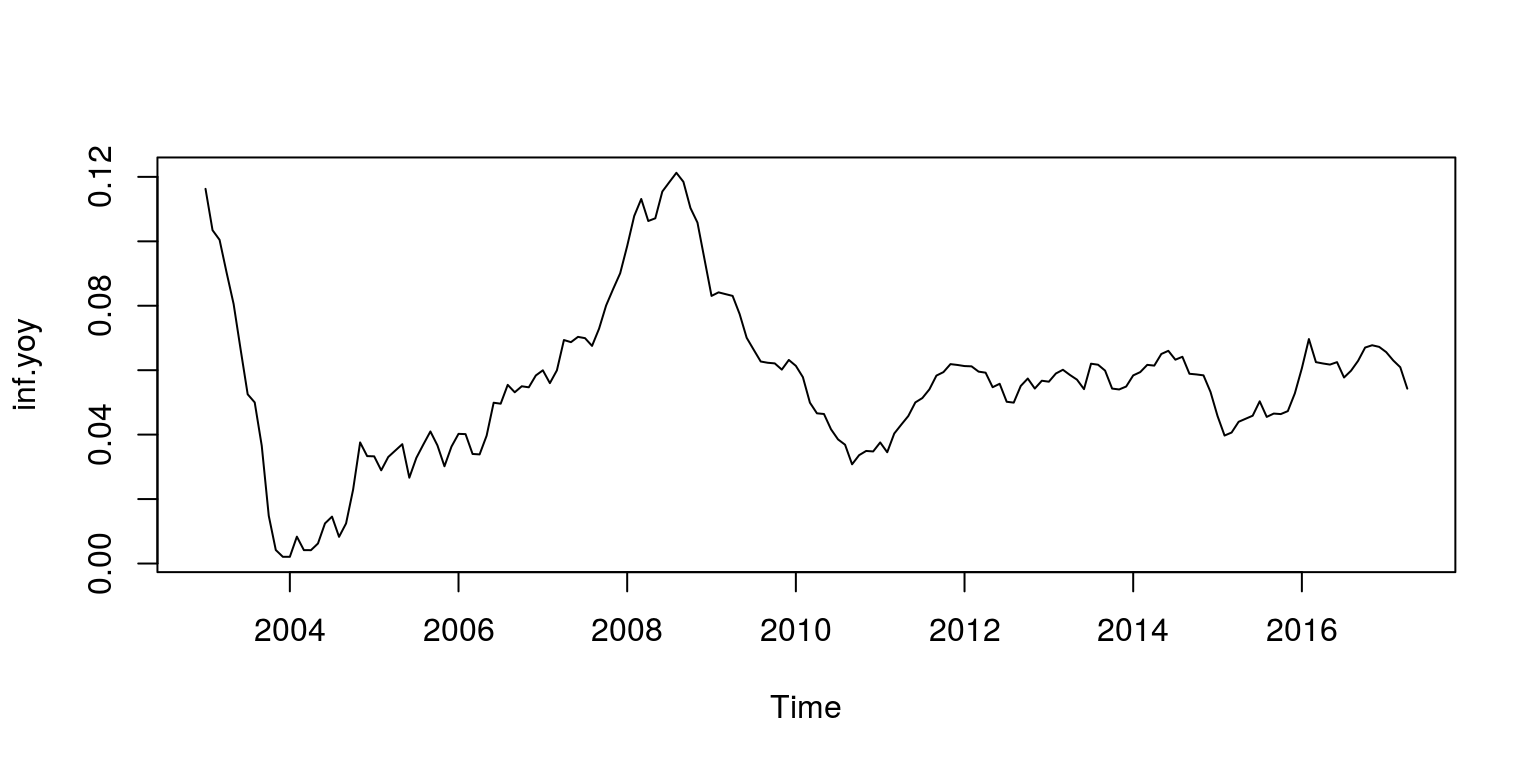As we are primarily interested in identifying the smoothed trend in this case, we will make use of a Daubechies function that is neither too smooth or sharp. Such a function would be the Daubechies 4 wavelet, that will be applied with the aid of the modified discrete wavelet transformation technqiue. In addition, we are also going to make use of three mother wavelets for the respective high frequency components.

``````inf.d4 <- modwt(inf.yoy, "d4", n.levels = 3)
names(inf.d4) <- c("w1", "w2", "w3", "v3")
inf.d4 <- phase.shift(inf.d4, "d4")``````

We can then plot the results for each of the separate frequency components as follows:

``````par(mfrow = c(5, 1))
plot.ts(inf.yoy, axes = FALSE, ylab = "actual", main = "")
for (i in 1:4) {
plot.ts(inf.d4[[i]], axes = FALSE, ylab = names(inf.d4)[i])
}
axis(side = 1, at = c(seq(1, (length(inf.yoy)), by = 48)),
labels = c("2002", "2006", "2010", "2014"))``````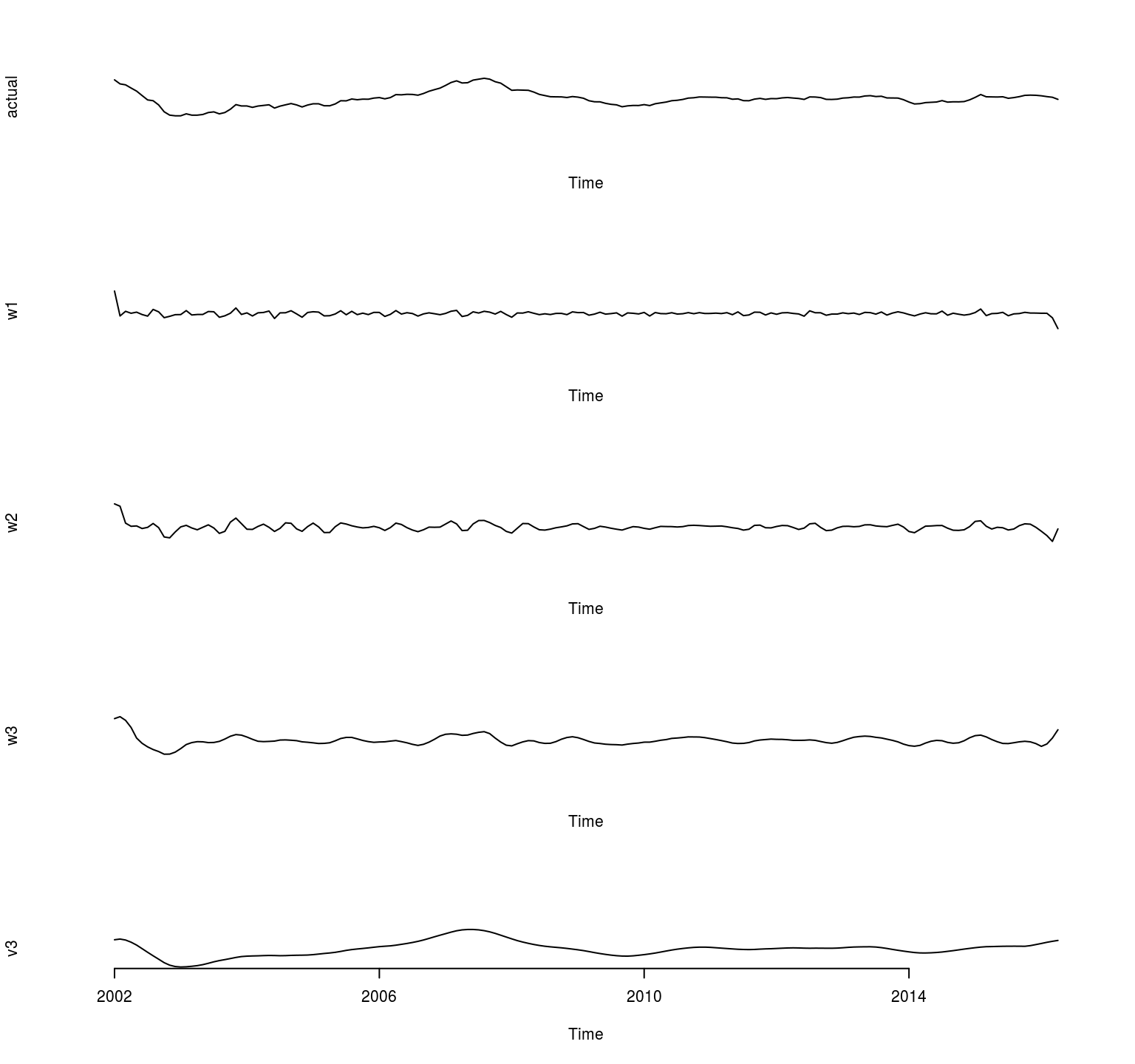If we now want to plot the trend (father wavelet) on the data.

``````inf.tren <- ts(inf.d4\$v3, start = c(2003, 1), frequency = 12)

plot.ts(inf.yoy, ylab = "inf")
lines(inf.tren, col = "red")``````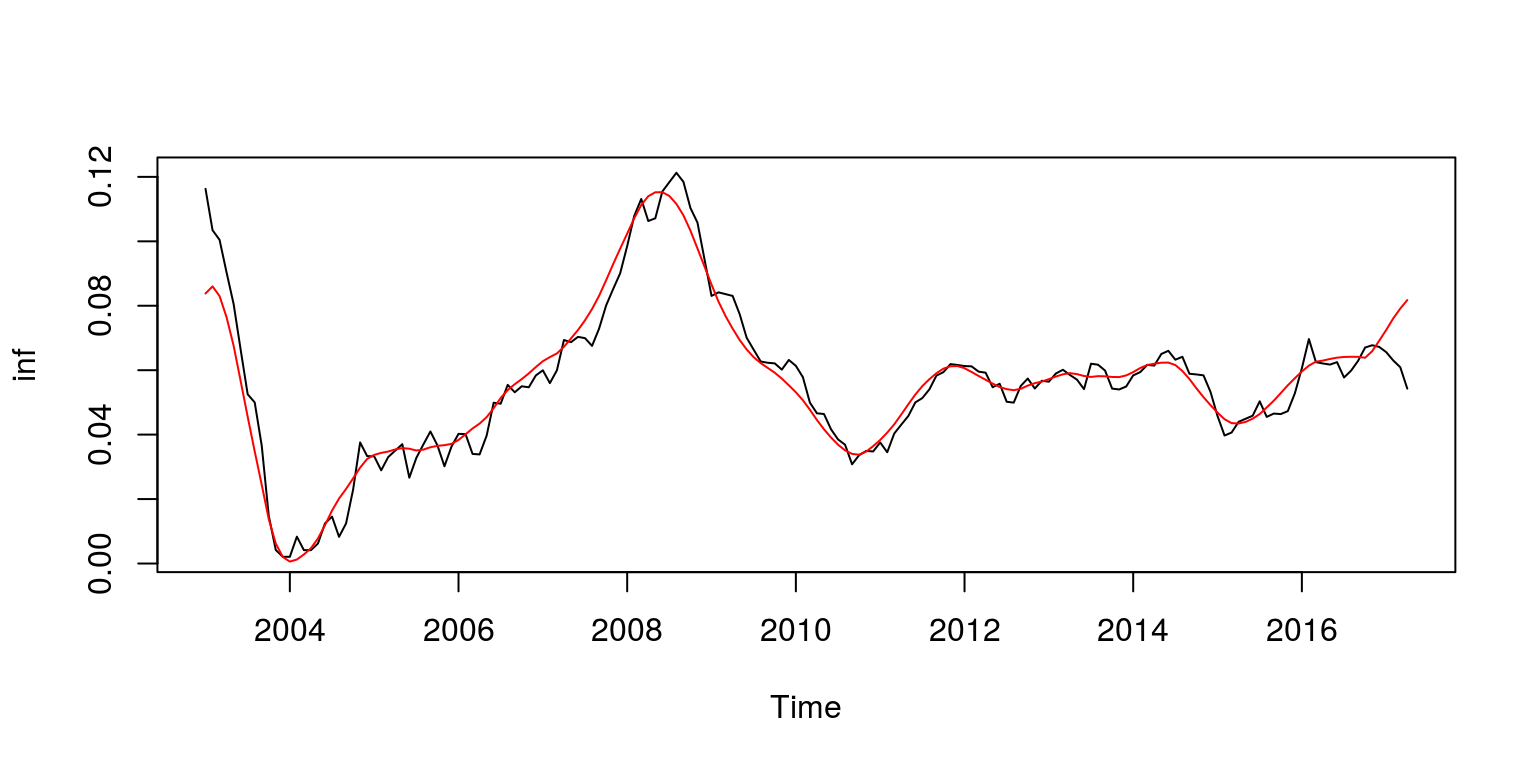Note that as the respective scales (or frequency bands) are additive, we could add one of the mother frequency bands to the trend as follows.

``````inf.tmp <- inf.tren + inf.d4\$w3
inf.tren2 <- ts(inf.tmp, start = c(2003, 1), frequency = 12)

plot.ts(inf.yoy, ylab = "inf")
lines(inf.tren2, col = "red")``````